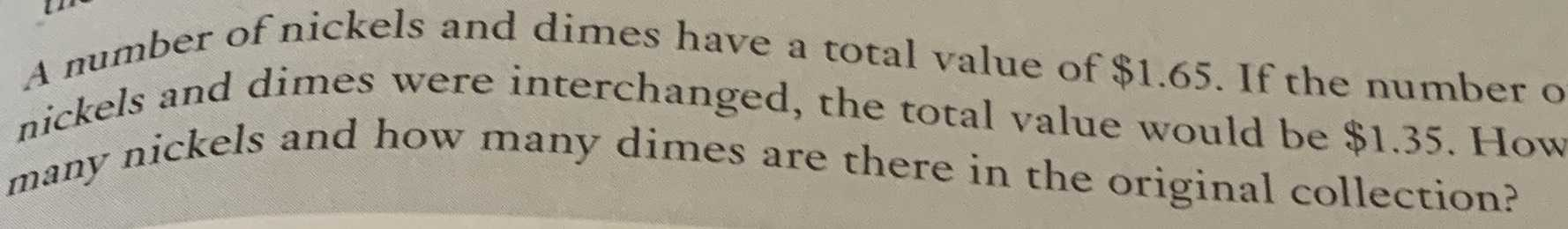### ¿Todavía tienes preguntas de matemáticas?

Pregunte a nuestros tutores expertos
Algebra
PreguntaA number of nickels and dimes have a total value of $$\ 1.65 .$$ If the number o nickels and dimes were interchanged, the total value would be $$\ 1.35$$ . How many nickels and how many dimes are there in the original collection?

$$0.1 x + 0.05 y = 1.65,0.1 y + 0.05 x = 1.35 \quad : \quad x = 13 , y = 7$$